# Education

Teaching quantum mechanics!

The nextnano software is an excellent tool for studying fundamental examples in Quantum Mechanics typically found in textbooks, making it an ideal resource for classroom instruction.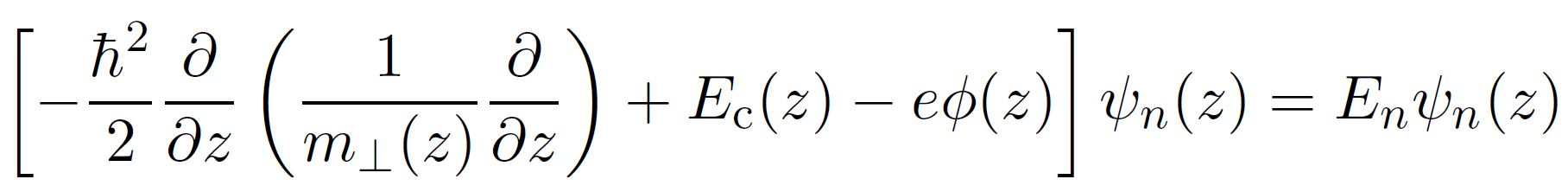## Examples

• Black body spectrum
• 1D, 2D and 3D Schrödinger equation for arbitrary potential energy profiles
• Infinite quantum well
• Finite quantum well
• Quantum harmonic oscillator
• Triangular quantum well
• Double quantum well
• Matrix elements and selection rules
• Expectation value
• Perturbation theory
• Tunneling: Transmission function of potential step, potential barrier, quantum well and double barrier
• Periodic structures: Superlattice
• Energy bands
• Band structure (Tight-binding)
• Many more...

A preliminary handout containing some nextnano examples is available here.

## Tight-binding (sp3s*)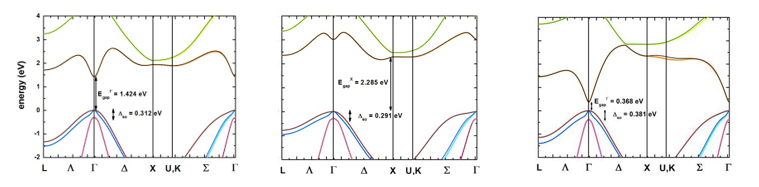What you can learn:
• tight-binding band structure of bulk semiconductors

## Dispersion in infinite superlattices: Minibands (Kronig-Penney model)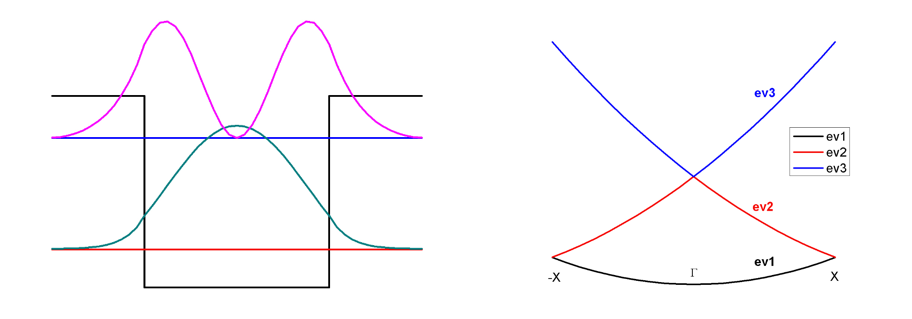What you can learn:
• Schrödinger equation in 1D with periodic boundary conditions
• superlattice dispersion
• band structure of an artificial crystal (quantum well crystal)

## Triangular quantum wellWhat you can learn:
• Energy levels and wave functions of a triangular quantum well
• Schrödinger equation

## Double quantum well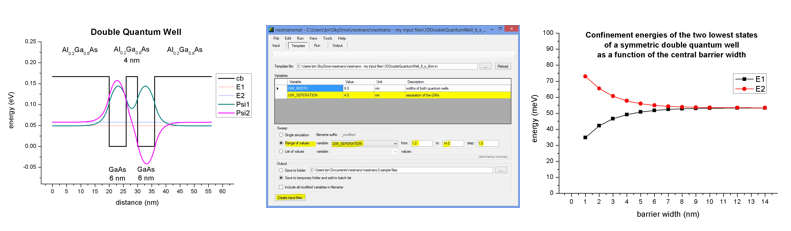What you can learn:
• Energy levels and wave functions of a double quantum well as a function of quantum well separation (barrier width)
• Schrödinger equation
• Template feature of nextnanomat

## Schottky barrier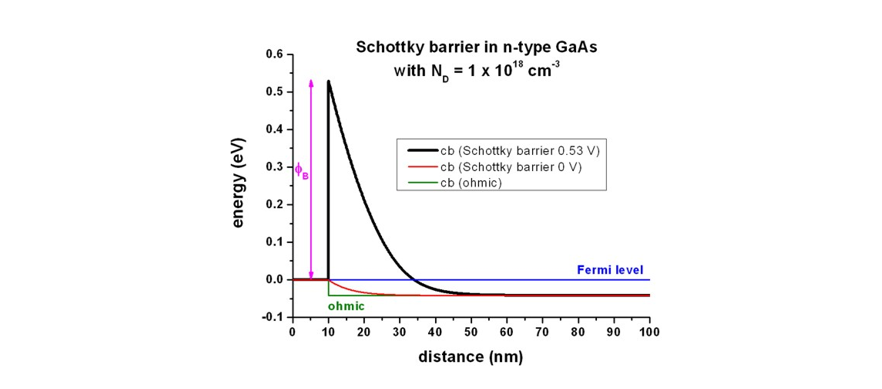What you can learn:
• Metal-semiconductor interface: Fermi-level pinning; Ohmic contact vs. Schottky contact vs. Surface charge density vs. Surface doping
• Poisson equation

## pn junctionWhat you can learn:
• doping
• Poisson equation
• apply a bias, drift-diffusion calculation, recombination
• Current-Poisson equation

## Quantum well (Interband transitions)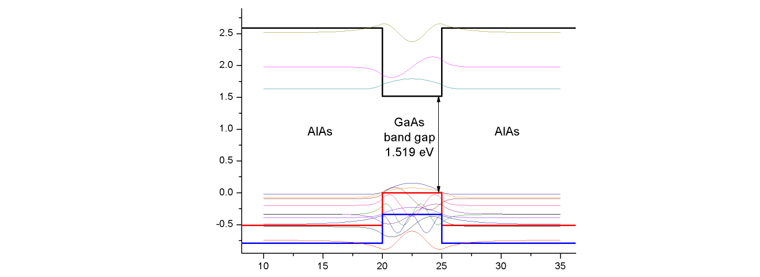What you can learn:
• Energy levels and wave functions of an infinite and finite quantum well, interband transition matrix elements (spatial wave function overlap), selection rules
• Schrödinger equation

## Spherical Si Quantum Dot (Artificial Atom)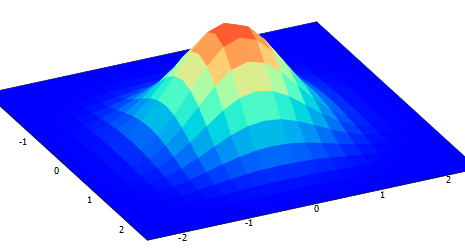What you can learn:
• 3D simulation, quantum dot, spin-orbit splitting, artificial atom
• 6-band k.p Schrödinger equation in 3D